#### Jackknife Method

The jackknife method of variance estimation deletes one PSU at a time from the full sample to create replicates. This method is also known as the delete-1 jackknife method because it deletes exactly one PSU in every replicate. The total number of replicates R is the same as the total number of PSUs. In each replicate, the sampling weights of the remaining PSUs are modified by the jackknife coefficient. The modified weights are called replicate weights.

Let PSU i in stratumbe omitted for the rth replicate; then the jackknife coefficient and replicate weights are computed asand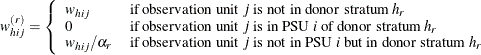You can use the VARMETHOD=JACKKNIFE(OUTJKCOEFS=) method-option to store the jackknife coefficients in a SAS data set and use the VARMETHOD=JACKKNIFE(OUTWEIGHTS=) method-option to store the replicate weights in a SAS data set.

If you provide your own replicate weights with a REPWEIGHTS statement, then you can also provide corresponding jackknife coefficients with the JKCOEFS= option. If you provide replicate weights with a REPWEIGHTS statement but do not provide jackknife coefficients, then the procedure uses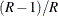as the default jackknife coefficient for every replicate, where R is the total number of replicates.

Let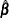be the estimated proportional hazards regression coefficients from the full sample, and let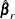be the estimated regression coefficients for the rth replicate. PROC SURVEYPHREG estimates the covariance matrix ofbywith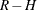degrees of freedom, where R is the number of replicates and H is the number of strata, or R – 1 when there is no stratification.

If you specify the CENTER=REPLICATES method-option, then PROC SURVEYPHREG computes the covariance matrix ofby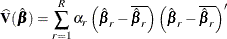whereis the average of the replicate estimates as follows: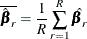If one or more components ofcannot be calculated for some replicates, then the variance estimator uses only the replicates for which the proportional hazards regression coefficients can be estimated. Estimability and nonconvergence are two common reasons whymight not be available for a replicate sample even ifis defined for the full sample. Let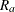be the number of replicates whereare available, and letbe the number of replicates whereare not available. Without loss of generality, assume thatis available only for the firstreplicates; then the jackknife variance estimator iswith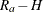degrees of freedom, where H is the number of strata. Alternatively, you can use the VADJUST=AVGREPSS option in the MODEL statement to use the average sum of squares for the invalid replicate samples. See Variance Adjustment Factors for details.